# Setting the stage for ASP functions

By
Pedro Cabalar Fernández
Department of Computer Science
University of Corunna, SPAIN

Abstract

In the recent years, several approaches for introducing evaluable, non-Herbrand functions in Answer Set Programming have been proposed. In this note we overview their different behaviours and features regarding their potential use, pointing out possible advantages and disadvantages together with future open topics and challenges.

### 1 Introduction

The usual view of functions in Logic Programming has been strongly influenced by one of the fundamental Prolog mechanisms, unification, and the corresponding semantic assumption of Herbrand interpretations. Under this perspective, function symbols act as syntactic constructors that allow forming objects in the universe, such as tuples or lists of elements, to put a pair of examples. Being constructors, it is natural that two syntactically different terms, such as vector(1, 2) and vector(2, 1), designate two different elements in the universe (in this case, for instance, two different vectors in a 2D coordinate system). Unfortunately, Prolog restriction to Herbrand functions has a cost from the representational point of view: functions in their usual mathematical meaning are not directly representable and must be simulated with predicates with an extra argument to capture the function value. For instance, the sum of two vectors cannot be represented as a function sum(vector(1, 2),vector(2, 1)) since, under Herbrand interpretations, it would be different from, say, sum(vector(1, 1),vector(2, 2)) although both expressions must be mapped to vector(3, 3) to get the intended meaning. The usual Prolog representation for this function would look like:

sum(vector(X,Y),vector(Z,T),vector(V,W)) :- V is X+Z, W is Y+T.

where sum(A,B,C) would correspond to sum(A,B) = C in functional notation. Although this difference may just seem syntactic sugar, the truth is that the functional notation would allow us building nested expressions that are much more comfortable from the representational point of view. For instance, this is so common for arithmetics, that Prolog provides a built-in predicate ‘is’ capable of evaluating a numeric expression with nested arithmetic operators, so that we can write a literal like:

X is Y+3*(Z-2*W)

which is clearly less readable and more prone to errors. Of course, we could also build a similar predicate isvec for evaluation of vector expressions, so that, for instance, we could write now a nested term like:

isvec(sum(vector(1,3),sum(vector(1,1),vector(2,2))), X)

to get the result in X. However, relying on this method means building a functional evaluation mechanism inside Prolog each time we deal with a family of evaluable functions. Think, for instance, what would happen if we wanted to evaluate an expression of the form:

sum(vector(age(John); age(Mary) + 10); vector(2; 3))
The need for dealing with evaluable functions (or operators) and combining them with Herbrand functions (or constructors) is part of the motivation of Functional Logic Programming (FLP) . For instance, a fragment1 of code in the FLP language Mercury could look like:

sum(vector(X,Y),vector(Z,T)) = vector(X+Z,Y+T).

which is a more readable and natural definition of the sum of vectors, and treats this operator in a more coherent way with respect to the sum of integers, for instance. FLP languages usually introduce further features from functional programming like type systems and higher order constructs, but these are out of the discourse of the current survey.

### 2 Herbrand Functions in ASP

In the case of Answer Set Programming (ASP), in the past, Herbrand functions have been traditionally considered a trouble to be avoided. The original definition of the stable model semantics  was thought for propositional programs: the use of variables was understood as a shorthand for their ground instantiations. As a consequence, the ASP paradigm has mostly inherited this orientation. ASP can be seen as a constraint-based problem solving paradigm  whose computation methods rely on two fundamental steps: a grounding phase that replaces variables by their possible instantiations and a solving phase that computes stable models or answer sets for the resulting ground program. The simple introduction of a single function symbol f would make the Herbrand universe infinite f(c),f(f(c)),f(f(f(c))),… and disable any direct attempt of obtaining a finite ground program. Thus, one of the distinctive features of ASP with respect to Prolog has traditionally been that function symbols were forbidden.

In the last years, however, this picture has changed in different ways. First, from the theoretical point of view, we have nowadays a general definition of stable model that covers any arbitrary first order theory, thanks to the definition of Quantified Equilibrium Logic (QEL) , a nonmonotonic formalism relying on a monotonic intermediate logic, or the equivalent General Theory of Stable Models , a syntactic construct very close to Circumscription . Under these extensions, we can even remove the restriction to Herbrand models, so that assumptions like, for instance, domain closure or unique names are now optional, at least at a theoretical level. Second, ASP grounders gradually accept a limited use of function symbols as constructors. For instance, grounders gringo and lparse allow using Herbrand functions with a limited nesting, something that, for instance, would allow us representing vectors with the construct vector(X,Y ), but not lists, since the latter require arbitrary nesting. The most significative breakthrough in this aspect has been the possibility of grounding ASP programs with arbitrarily nested Herbrand functions  and its implementation with solver DLV-complex . When a program with functions, disjunction and negation satisfies a given property, so-called being finitely-ground, it has nice computational features: brave and cautious reasoning become decidable, and its answer sets are computable. An interesting result is that finitely-ground programs can encode any computable function. As it can be expected, however, checking whether a program is finitely recursive is undecidable.

### 3 Evaluable Functions in ASP

While these advances in the interpretation and implementation of Herbrand functions have made ASP fully comparable to Prolog in this aspect, in the case of evaluable functions, the state of the art in ASP is at a preliminary stage, still far away from the situation we find in FLP, for instance. Even so, the recent bibliography has shown an increasing interest in the topic with the constant inclusion of contributions related to non-Herbrand functions in the main conferences related to ASP. In this preliminary stage, most efforts have been focused on establishing a suitable semantic definition for this kind of functions, although several implementations are already available. Unfortunately in this case, the amount of contributions is not meaning a similar increase in scientific progress due to the lack of agreement in the understanding and expected behavior of evaluable functions. While Herbrand functions do not leave much choices for their semantic treatment (after all, they are a syntactic concept), evaluable functions can be understood in many different ways. In the last five years there have been five different proposals and we are only beginning to understand their formal relations, correspondences and differences in behavior. We will discuss next each proposal by chronological ordering, trying to summarize their main features, and potentially strong and weak points.

#### 3.1 Lin and Wang’s rigid functions

The first of the approaches we consider was introduced by Lin and Wang in . In this approach functions are complete (or total) in the sense that any term f(x) is always associated some value in the universe. Furthermore, the evaluation of a function is what we can call rigid knowledge. Let us explain this in more detail. For the syntactic class of logic programs, all the approaches can be formulated in terms of a program reduct, a syntactic transformation in the spirit of the original reduct construction by Gelfond and Lifschitz . Formally, if we have an interpretation I (or assumption), candidate model of a program Π, we define a program reduct ΠI by some transformation on Π that (among other things) removes default negation. Then we choose some selected model(s) from ΠI and, to form a stable model, we check that one of them coincides with the initial assumption.

Lin and Wang’s functions are “rigid” in the sense that if we take an interpretation I to build the reduct ΠI, then the models of ΠI also use the interpretation of functions fixed by I. So, there is no variability in the interpretation of functions when deciding whether I is a stable model or not.

Lin and Wang’s formalism is a many-sorted first order language, so that all constants, variables, predicate arguments, function arguments and values belong to a predefined type or sort, containing a finite and non-empty set of elements. As an example, the following would be an encoding of the Hamiltonian cycle problem: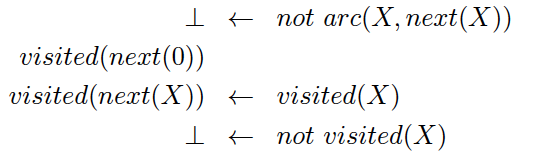where each Hamiltonian cycle in the graph corresponds to a stable model. The cycle is encoded by function next(X) saying which is the next node of X in the path. The rules above would be accompanied by the following type and sort definitions: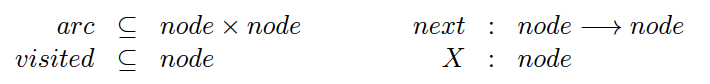One of the main advantages of Lin and Wang’s approach is its simplicity. Since functions are somehow “external” to the model minimization process, their implementation using an external solver for Constraint Satisfaction Problems (CSP) is relatively simple2. In fact, Lin and Wang presented a tool called FASP that relied on a constraint solver backend and showed how it resulted competitive with non-functional ASP encodings for problems involving functional dependencies. In particular, in those domains, the use of functions allowed much smaller results in the grounding phase although, of course, the computed results are not directly comparable, since FASP grounding yields a CSP problem with finite domain variables rather than a ground ASP program with propositional atoms.

From a logical point of view, it was proved in  that Lin and Wang’s approach corresponds exactly to Quantified Equilibrium Logic with static domains and Herbrand interpretations, which was actually the way in which domains and functions were treated in the original definition of QEL . Thus, in a sense, FASP can be considered a sorted implementation of the original semantics presented in .

Perhaps a brief explanation about QEL may help us to understand the logical meaning of rigid total functions. QEL relies on a monotonic intermediate logic called Quantified Here-and-There (QHT) (a first order extension of Heyting’s logic of Here-and-There ). Interpretations in QHT have the form ⟨D,σ,Ih,It⟩ where D is the universe or set of elements, σ is a mapping from terms to elements in D, and Ih,It respectively called worlds here and there, are sets of ground atoms for predicates satisfying Ih ⊆ It. Intuitively, It informally corresponds to candidate interpretations used to build the reduct ΠIt whereas I h would be each possible model of that reduct. An equilibrium model must satisfy Ih = It and that Ih is minimal fixing It. When ⟨D,σ,It,It⟩ is an equilibrium model, we say that the first order interpretation ⟨D,σ,It⟩ is a stable model. Under this setting we say that interpretations are static in the sense that D and σ are common for worlds here and there (in fact, this is a standard terminology in intuitionistic and intermediate logics).

The main disadvantage of rigid functions is that they cannot be used for non-monotonic reasoning (NMR). In particular, there is no way of defining a function default value without resorting to predicate-based representations of functions, so that part of the representational advantages of functions would be lost. Note that function default values are crucial, for instance, if we want to deal with a functional fluent. For instance, take the location of a block loc(B) in the Blocks World domain. We would need to specify its inertia law informally as follows: loc(B) at state i + 1 should have by default the same value as in state i unless we can find evidence of a change to a different value.

Generally speaking, if we plan to use evaluable functions for NMR, one would expect that functions alone should be capable of capturing full ASP by the following simple translation. For each ASP atom p we would define a 0-ary function with the same name p that ranges in the Boolean domain {true,false}. Then, we should be able to specify in some way that the function default value is false. Finally, we would replace each ASP positive literal p by fp = true and each negative literal not p by p = false. As an example, the following ASP ground program: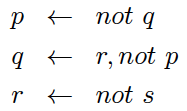would be re-encoded using this technique as the functional logic program: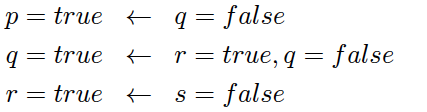Since Lin and Wang’s functions cannot deal with default values, the above translation in this case would collapse to classical propositional logic (i.e., stable models in FASP would just correspond to the classical models of the original ASP ground program).

#### 3.2 Cabalar’s partial functions

The idea of functions with default values had been explored in the past by Cabalar and Lorenzo  although their formalism was not a proper extension of stable models, since it did not considered default negation. In , Cabalar introduced such an extension under the name of QELF . This formalism has two main differences with respect to Lin and Wang’s functions:

1. functions can be partial, that is, a function f(x) may have no designated value and be left undefined
2. functions are not rigid but flexible instead, that is, we can vary their meaning when deciding whether an interpretation I is a stable model.

The definition of QELF is a variant of QEL where interpretations have the form now ⟨D,σh,Iht,It⟩, that is, we have two differentiated mappings σ for assigning domain elements to terms. These mappings can now be partial but must respect the condition that if σh(x) is defined, then the interpretation “there” coincides σt(x) = σh(x). This follows the intuitionistic principle of knowledge persistence as in the the analogous condition Ih ⊆ It for sets of atoms.

Note that features (i) and (ii) are actually independent. We can have partial functions that are rigid: this would mean that we cannot specify default values for them, but they can be left undefined. A clear example of this would be, for instance, the division div(x,y) that must be undefined when y = 0 but whose interpretation is predefined in any context and has not any particular default value. To force a function to become rigid in QELF we would include the excluded middle axiom: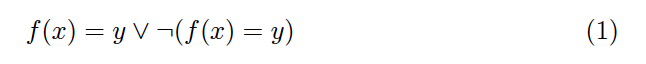assuming that x and y are universally quantified and that ¬ stands for default negation. We can also fix total functions with flexible interpretation. In this way, a function would always be total in the stable models, but for deciding whether some particular interpretation I is a stable or equilibrium model, we could vary functions in σh by making them partial. In other words, minimal models of the reduct ΠIt could leave some functions undefined. As an example, consider the program: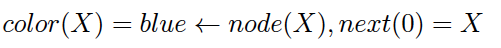where blue is a Herbrand constant. Intuitively, this means that the next node of 0 will be assigned color blue. When we allow partial functions, color(X) will be left undefined for any element that is not the next node of 0. If we add a constraint of the form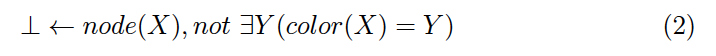for any element X this intuitively means that all nodes in a stable model must have some defined color3. Then, the resulting program has no stable models, since for any node X that is not the next of 0, no matter the color Y we choose for color(X) = Y we will always have a smaller model where color(X) is left undefined. In other words, there is no evidence in the program to “complete” the information about the color values for the rest of nodes.

In  it was shown that, by removing (i) and (ii) with axioms like (2) and (1) respectively, we actually obtain Lin and Wang’s rigid functions.

Suppose that we want to represent instead that all nodes have color red by default. This could be expressed with the rule: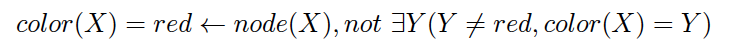or in an abbreviated representation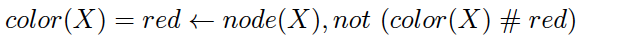where the ‘#’ operator is a kind of difference expressing that the function value exists, but is different from red.

Similarly, as an example of inertia rule, we could have for instance: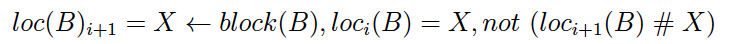Although as it can be imagined, this is the author’s preferred approach, probably the main objection to Cabalar’s definition when compared to the others’ is the technical difficulty derived from handling partial functions. As explained in  this difficulty affects to the interpretation of equality and requires some special syntactic constructs to properly exploit nested functional terms in logic programs. To understand some of the possible issues that may arise, consider for instance a theory containing the single atom: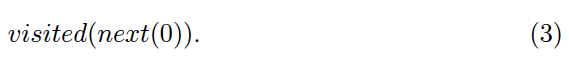Since we have not provided information about next(0) we would expect a unique stable model with next(0) undefined and visited(X) false for any element X. However, forcing the truth of an atom means that its arguments must be defined and thus, the program above has no stable models. In practice, logic program rules must be translated by reinforcing the rule body with definedness conditions for all terms in the head. Thus, when (3) is understood as a program fact, it is actually translated as the formula: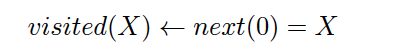so that next(0) = X in the body imposes the condition that next(0) is defined with value X. The definitions in  were implemented in a system called lppf that deals with partial functions and accepts nested functional terms.

#### 3.3 Lifschitz’s intensional functions

In , Lifschitz introduced a third approach for functions in ASP called Intensional Functions (IF). In this formalism, functions are total and flexible, but unlike QELF , are never left undefined. In this way, when we consider models of the program reduct ΠI we may make an interpretation of functions different from the one in I, but still total. To stress the difference between QELF and IF suppose that I fixes the assignment color(1) = blue. Then, in QELF models of ΠI could either make color(1) = blue or leave color(1) undefined. In IF, models of ΠI could assign any value (not necessarily blue) such as color(1) = red, for instance, but will never leave color(1) undefined.

The original motivation for Lifschitz’s approach has a close connection to the idea of causal justification (inspired by McCain and Turner’s causal logic ). Informally speaking, any function value in a stable model must be justified in the sense that no other value would make true the “same” theory. When compared to the usual stable model condition, rather than checking that a candidate interpretation I is minimal among models of ΠI, IF requires instead that I is the only model of ΠI (regarding interpretation of functions).

One of the nicest features of IF is the simple and intuitive representation we can get for a function’s default value. The formula: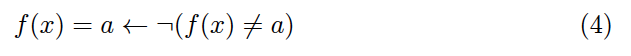means that the default value for f(x) is a. In fact, the reading of the formula expresses this idea in a straightforward manner: f(x) is a if we cannot prove that it is different from a.

The main disadvantage of IF is that it is closer to non-monotonic causal logic than to the behavior that one would expect from logic programming under the stable models semantics. The following are a pair of examples extracted from  whose IF semantics is counter-inutitive from the ASP point of view. The program Π1: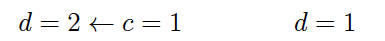has an IF-stable model I where I(c) = 2 and I(d) = 1 but there is no evidence in the program for assigning I(c) = 2. As a second example, the disjunctive program Π2: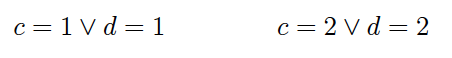has no IF-stable models whereas, if we thought of equalities above as ground atoms, one would probably expect to get two stable models, one with c = 1,d = 2 and the other with d = 1,c = 2.

One final remark confirming that IF is an approach less close to ASP is the fact that its formulation in analogous terms to equilibrium logic requires abandoning the monotonic basis of the logic of Here-and-There and replacing it instead by a different formalism called Bi-state logic .

#### 3.4 Bartholomew and Lee’s intensional functions

In , Bartholomew and Lee introduced a reformulation of intensional functions (we will call BL) that keeps the main functional properties of IF, but provides a closer behavior to ASP. As in IF, functions are flexible and always total, but in BL, the model minimization condition is taken up again instead of requiring an unique causal explanation.

Without entering into formal details, BL keeps the nice representational properties of IF such as the natural formulation of default values as in (4). On the other hand, programs like Π1 and Π2 seen before have a closer interpretation to ASP. For instance, Π1 has no stable model, since we have no evidence in the program to fix any value for c. Note that this lack of information in QELF would be accommodated in a different way, yielding a unique stable model with c undefined and d = 1. Example Π2 in BL yields the two expected stable models corresponding to {d = 1,c = 2} and {c = 1,d = 2}.

From a logical point of view, it was recently (and independently) shown in  and  that BL corresponds to a variant of Quantified Equilibrium Logic that allows flexible total functions. In fact,  contains a detailed formal comparison between BL and QELF that has clarified the picture, showing relevant differences, but also an important syntactic class where both semantics coincide (under the assumption of total functions). In particular, Bartholomew and Lee define a class of theories for which BL and QELF coincide: these are called c-plain theories and correspond to the absence of nested functions or equalities between functions. This is an important result since, as shown in  for logic programs and later in  for any arbitrary theory, it is always possible to reduce a formula in QELF to a strongly equivalent expression in c-plain form. In other words, we can always “unfold” nested functions in QELF . To put an example, the formula f(g(x),y) = h(y) with functions f,g,h can be reformulated by introducing auxiliary variables as ∃z,t(g(x) = z ∧ h(y) = t ∧ f(z,y) = t). This mechanism is well known in Functional Logic Programming (it has received the name of “flattening” ) and was the basic technique used in system lppf to transform functional logic programs into regular ASP.

Unfortunately, function unfolding in BL does not preserve strong equivalence in the general case. An example extracted from  is the simple formula f = g comparing two 0-ary functions. In QELF this formula is strongly equivalent to ∃x,y(f = x∧g = y ∧x = y) but in BL no. For instance, if we take a universe with the three elements {1, 2, 3}, f = g would have no BL-stable models, whereas its unfolding has three BL-stable models with f = g = 1, f = g = 2 and f = g = 3 respectively. As we explained before, the latter is the meaning assigned by QELF for both formulas.

The question whether c-plain formulas constitute a normal form in BL, as does happen in QELF , is still an open problem. There could perhaps exist a different syntactic transformation that may allow us to obtain, from an arbitrary formula with nested functions, a BL-strongly equivalent c-plain formula. Finding such a transformation is interesting because it may also help to understand the meaning of nested functions in this semantics.

#### 3.5 Balduccini’s partial functions

The last approach to go on stage was presented by Balduccini in  and consisted in a combination of partial functions with strong negation. The main motivation of this approach was obtaining an efficient implementation , called ASP{f}, that was able exploit functional dependences to save grounding effort. This was an important result, since it obtained an improvement comparable to Lin and Wang’s FASP system, but allowing partial functions and using an ASP solver as a backend.

However, as recently proved in , Balduccini’s approach does not mean a significative innovation with respect to QELF . In fact, when strong negation is not involved, both semantics coincide (in the restricted syntactic fragment for which Balduccini’s semantics is defined). Furthermore,  also showed that strong negation can be encoded as a derived operator in QELF .

### 4 Conclusions

In this informal survey, we have reviewed the main current approaches for interpreting evaluable functions in ASP. As a summary, QELF deals with flexible, partial functions and generalizes both Lin and Wang’s rigid functions and Balduccini’s ASP{f} language. In fact, the latter can be understood as an implementation of QELF for a syntactic subset. On the other hand, Bartholomew and Lee’s (total) intensional functions (BL) are close to Lifschitz’s approach in its simplicity but, at the same time, improve some counterintuitive results from the latter (when understood from an ASP perspective). Finally, QELF and BL have the same behavior when functions are not nested or compared using equality, but differ in the general case.

The most important open topic for the immediate future is reaching an agreement on a suitable interpretation of evaluable functions and extending the current prototype systems to fully operative tools or to language extensions in the main ASP solvers.

### References

   Marcello Balduccini. An answer set solver for non-herbrand programs: Progress report. In ICLP Technical Communications), pages 49–60, 2012.

   Marcello Balduccini. A “conservative” approach to extending answer set programming with nonherbrand functions. In Esra Erdem, Joohyung Lee, Yuliya Lierler, and David Pearce, editors, Correct Reasoning, pages 24–39. Springer-Verlag, 2012.

   Michael Bartholomew and Joohyung Lee. Stable models of formulas with intensional functions. In Proceedings of International Conference on Principles of Knowledge Representation and Reasoning (KR’12), pages 2–12, 2012.

   Luis Fariñas del Cerro, David Pearce and Agustín Valverde.  FQHT: The logic of stable models for logic programs with intensional functions. In Proceedings of International Joint Conference on Artificial Intelligence (IJCAI’13), 2013. (to appear).

   Michael Bartholomew and Joohyung Lee. On the stable model semantics for intensional functions. In Proceedings of International Conference on Logic Programming (ICLP’13), 2013. (to appear).

   Pedro Cabalar. Existential quantifiers in the rule body. In Proc. of the 23rd Workshop on (Constraint) Logic Programming (WLP’09), 2009.

   Pedro Cabalar. Functional answer set programming. Theory and Practice of Logic Programming, 10(2-3):203–233, 2011.

   Pedro Cabalar and David Lorenzo. Logic programs with functions and default values. In Proc. of the 9th European Conf. on Logics in AI (JELIA’04) (LNCS 3229), pages 294–306, 2004.

   Francesco Calimeri, Susanna Cozza, Giovambattista Ianni, and Nicola Leone. Computable functions in ASP: Theory and implementation. In 24th Intl. Conf. on Logic Programming, volume 5366 of Lecture Notes in Computer Science, pages 407–424. Springer-Verlag, 2008.

   Francesco Calimeri, Susanna Cozza, Giovambattista Ianni, and Nicola Leone. An ASP system with functions, lists, and sets. In 10th Intl. Conf. on Logic Programming and Nonmonotonic Reasoning, volume 5753 of Lecture Notes in Computer Science, pages 483–489. Springer-Verlag, 2009.

   Luis Fariñas del Cerro, David Pearce, and Agustín Valverde. Bi-state logic. In Esra Erdem, Joohyung Lee, Yuliya Lierler, and David Pearce, editors, Correct Reasoning, pages 265–278. Springer-Verlag, 2012.

   P. Ferraris, J. Lee, and V. Lifschitz. A new perspective on stable models. In Proc. of the International Joint Conference on Artificial Intelligence (IJCAI’07), pages 372–379, 2007.

   Michael Gelfond and Vladimir Lifschitz. The stable model semantics for logic programming. In Proc. of the 5th Intl. Conf. on Logic Programming, pages 1070–1080, 1988.

   Michael Hanus. The integration of functions into logic programming: from theory to practice. Journal of Logic Programming, 19,20:583–628, 1994.

   Arend Heyting. Die formalen Regeln der intuitionistischen Logik. Sitzungsberichte der Preussischen Akademie der Wissenschaften, Physikalisch-mathematische Klasse, pages 42–56, 1930.

   Joohyung Lee and Ravi Palla. System F2LP – computing answer sets of first-order formulas. In Proc. of the 10th Intl. Conf. on Logic Programming and Nonmonotonic Reasoning (LPNMR’09), pages 515–521, 2009. Lecture Notes in Artificial Intelligence 5753.

   Vladimir Lifschitz. Logic programs with intensional functions. In Proceedings of International Conference on Principles of Knowledge Representation and Reasoning (KR’12), 2012.

   F. Lin and Y. Zhao. ASSAT: Computing answer sets of a logic program by sat solvers. Artificial Intelligence, 157(1-2):115–137, 2004.

   Fangzhen Lin and Yisong Wang. Answer set programming with functions. In Proc. of the 11th Intl. Conf. on Principles of Knowledge Representation and Reasoning (KR’08), 2008.

   V. Marek and M. Truszczyński. Stable models and an alternative logic programming paradigm. In The Logic Programming Paradigm: a 25-Year Perspective, pages 169–181. Springer-Verlag, 1999.

   Norman McCain and Hudson Turner. Causal theories of action and change. In Proceedings of the National Conference on Artificial Intelligence (AAAI’97), pages 460–465, 1997.

   J. McCarthy. Circumscription: A form of non-monotonic reasoning. Artificial Intelligence, 13:27–39, 1980.

   Lee Naish. Adding equations to NU-Prolog. In Proc. of the 3rd Intl. Symp. on Programming Language Implementation and Logic Programming, number 528 in LNCS, pages 15–26. Springer-Verlag, 1991.

   I. Niemelä. Logic programs with stable model semantics as a constraint programming paradigm. Annals of Mathematics and Artificial Intelligence, 25:241–273, 1999.

   David Pearce and Agustín Valverde. Towards a first order equilibrium logic for nonmonotonic reasoning. In Proc. of the 9th European Conf. on Logics in AI (JELIA’04), pages 147–160, 2004.

   Céline Rouveirol. Flattening and saturation: Two representation changes for generalization. Machine Learning, 14(1):219–232, 1994.